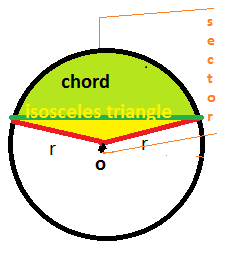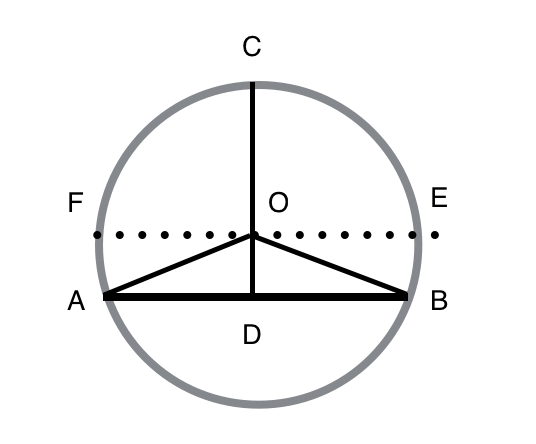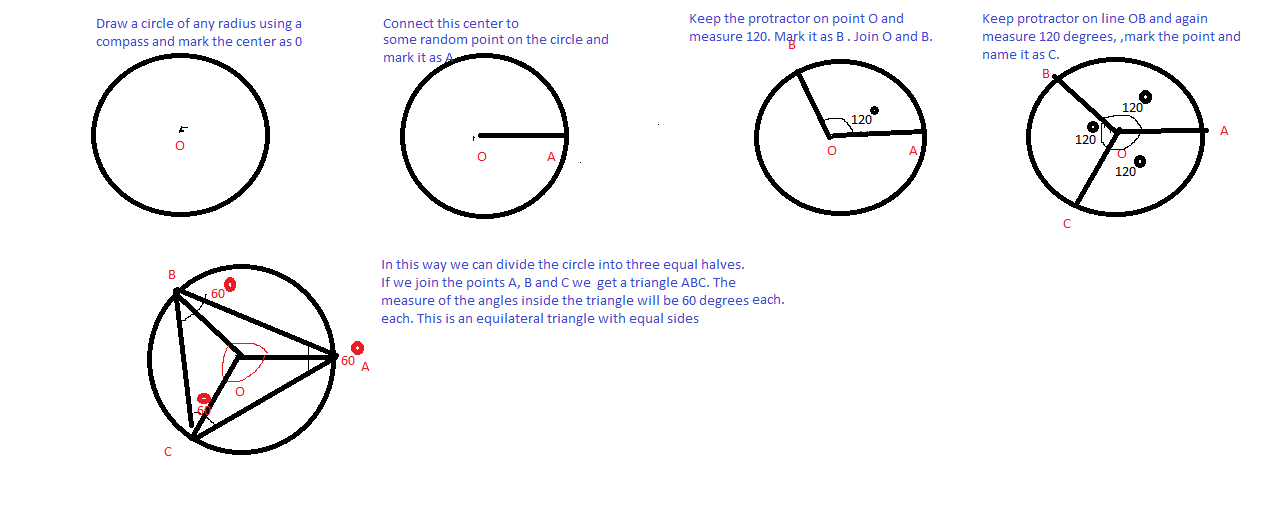D

#### Dividing circle into geometrically different thirds

57 viewed last edited 2 years agoDeepika Visala
0
A question that I was recently thinking of and couldn't work out an answer to was to determine a general solution as to the size of the shapes needed to make a circle divided into equal size parts using this shape: http://imgur.com/a/0Z5t0 where the white dot in the middle is the centre of the circle. Thanks :)Krishna
1Given in the question The size of the shapes needed to make a circle divided into equal size parts using this shape: We have to determine the three equal parts. So we need to imagine chords on the circle that divides the circle area into three equal parts. The area of the each part is must be \frac{1}{3}. First, we have to find out the area of the one part under the chord. Remaining are similar to this. We don,t have a direct formula for the area under the chord. but we know the formula of 1) The area of a sector in terms of \Theta. A = \frac{r^2 ( \theta)}{2} 2)The area of the isosceles triangle within the circular sector. A = \frac{r^2 * \sin (\theta)}{2} See the figure: chord area( \frac{\pi * r^2}{3}) = (the area of the sector) - (the area of the isosceles triangle ) \frac{\pi * r^2}{3} = \frac{r^2 * \theta}{2} - \frac{r^2 * \sin (\theta)}{2} \frac{\pi * r^2}{3} = \frac{r^2 }{2}(\theta -\sin (\theta) 2(\pi ) = 3*(\theta -\sin (\theta) f(\theta) = 3*\theta -3\sin (\theta) - 2* \pi Clearly, must be between zero and \pi radians, so using those as the initial boundaries, I quickly divided the range of possible values of in half and zeroed in on the approximate value of that answers your question. f(0) = -2(3.14) f(3.14) = 3(3.14) - 3 sin (3.14) - 2 (3.14) = 3.13522 f(2.6053) = 3(2.6053) - 3sin (2.6053) - 2(3.14) = 0.0003657 verify for the numbers in between the 0 to 3.14 \theta = 2.6053 a circular segment subtended by an angle of 2.6053 radians has an area of one third of the area of the circle itself. The length of the chord can be calculated given the radius r and this angle \theta using simple triangle.Mahesh Godavarti
0
Do you have a Geometric method that accomplishes this?Vivekanand Vellanki
1In the attached figure, FE is parallel to AB. Let, the angle OAD be \theta degrees. It is also known that \theta < 30 since if angle OAD is 30, the area of the sector AOC will be 1/3rd the area of the circle. The area of ADC is given to the 1/3 area of the circle. Assuming unit circle, Area of ADC = Area of sector AOC + area of triangle AOD. Area of sector AOC = \pi\frac{90+\theta}{360} To find area of triangle AOD. OD=\cos{\theta} and AD=\sin{\theta} Area of triangle AOD = \frac{\cos{\theta}\sin{\theta}}{2} This gives the equation: \pi\frac{90+\theta}{360} + \frac{\cos{\theta}\sin{\theta}}{2} = \pi/3 \frac{\cos{\theta}\sin{\theta}}{2} = \pi(1/3 - \frac{90+\theta}{360}) \frac{\cos{\theta}\sin{\theta}}{2} = \pi\frac{30 - \theta}{360} \cos{\theta}\sin{\theta} = \pi\frac{30 - \theta}{180}Mahesh Godavarti
0
Do you have a geometric construction for this?Sangeetha Pulapaka
0Draw a circle of any radius and mark the center of the circle as O. Mark some random point on the circumference of the circle and name it as A. Join OA. Keep the protractor on line OA and measure 120 ^\circand mark it as point B. Now keep the protractor on line OB and measure 120 ^\circ again, this time mark the next point as C.The circle is divided this way into three equal parts. Join the points ABC to get an equilateral triangle with angles 60 ^\circ each.This means that all the three sides are of equal length.